## Summary

In the last tutorial we learned how to generate random numbers in a shader. In this one we’ll go into interpolating between random numbers to generate noise that’s smoother and gradually changes. Because we need random values to interpolate between for value noise, you should know how to generate random values in shaders before doing this tutorial. Value noise is similar to perlin noise, but different because we always interpolate between the center of the cells, perlin noise will be explained in a later tutorial.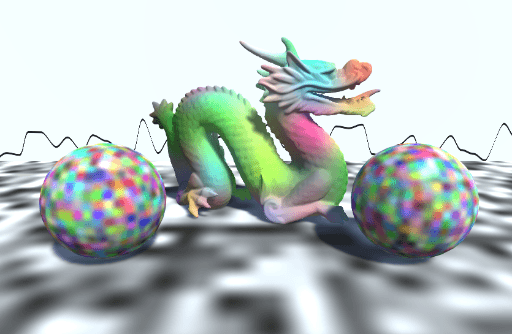## Show a Line

First we will implement an easy way for us to visualize 1d noise. To do that we start with the noise with cells of the previous tutorial and expand from there. We then change the cell size to a float value because we’ll operate in 1d for now. Then we’ll also make the value we feed to our noise function scalar by only using the x component of the position and use the 1d to 1d random function.

``````Properties {
_CellSize ("Cell Size", Range(0, 1)) = 1
}
``````
``````float _CellSize;
``````
``````void surf (Input i, inout SurfaceOutputStandard o) {
float value = floor(i.worldPos.x / _CellSize);
o.Albedo = rand1dTo1d(value);
}
``````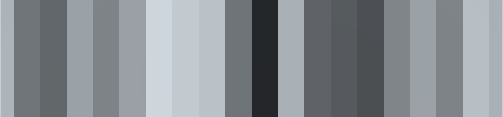With those changes we can now see the scalar values we generate as greyscale values. But to see how the values change even better, we’ll change that to a line. For that we first calculate the distance in the y direction of each pixel to the random value of it’s x position. We could also try to calculate the closest point on the line in general, but that woule be way more complex and we don’t need it for our cause. We get the distance from the line by simply subtracting our y position from the noise value and then taking the absolute value of that.

``````void surf (Input i, inout SurfaceOutputStandard o) {
float value = floor(i.worldPos.x / _CellSize);
float noise = rand1dTo1d(value);
float dist = abs(noise - i.worldPos.y);
o.Albedo = dist;
}
``````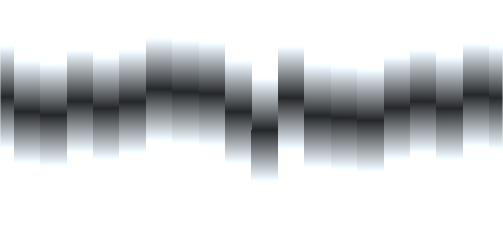Then we can use this distance to cut off the value so that we get a thin line. A nice way to generate a 1px thick line is to calculate how much the value we’re using changes in the neighboring pixels. The function to get that value is called `fwidth`, it automatically compares the neighboring pixels and returns approximately how much the value changes (also known as partial derivative magnitude), the reason that’s possible is that in the shader the fragments are handled in tiny 2x2 units so the fragment shaders running in paralell can compare their values. In our case we care about how much the y part of our position changes in the neighboring pixels, so we just put that value in the function. Then we do a smoothstep, the first value is the value that’s going to represent the 0(black) output value, so in our case the very center of the line, `0`, then the second value will represent at which value the function will return 1 (white) and then the third value is the value we compare the first two to. So when the third value is 0, the function will return 0 and for values of the pixel height or higher it will return white, giving us a nice thin line at all resultions.

``````void surf (Input i, inout SurfaceOutputStandard o) {
float value = floor(i.worldPos.x / _CellSize);
float noise = rand1dTo1d(value);
float dist = abs(noise - i.worldPos.y);
float pixelHeight = fwidth(i.worldPos.y);
float lineIntensity = smoothstep(0, pixelHeight, dist);
o.Albedo = lineIntensity;
}
``````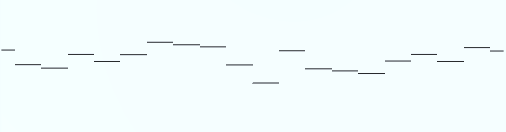## Interpolate Cells in one Dimension

To interpolate between the cells, we first have to sample the noise twice per fragment. Once in the cell we come from, and once in the cell we’re going to. We do this by only calculating the value with the cell size factored in at the start without flooring it. We then floor it for the “previous cell” value and we ceil it to get the “next cell” position. For the interpolation between the values we can simply use the fractional part of the value to interpolate between the cell values. 0 means we completely use the value of the previous cell, 1 means we’ll use the value of the next cell and the values between are interpolated.

``````float value = i.worldPos.x / _CellSize;
float previousCellNoise = rand1dTo1d(floor(value));
float nextCellNoise = rand1dTo1d(ceil(value));
float noise = lerp(previousCellNoise, nextCellNoise, frac(value));
``````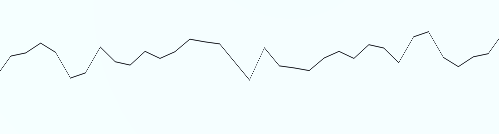This already gives us a connected line, but I’d like to make it softer. For this we’ll write a simple easing function, I’ll get more into easing functions in a later tutorial, but a simple one will be enough for now. First we do the easing in part of the function. for that we’ll simply use a quadratic function, that way the edge cases of our interpolation where our interpolation value has the value 0/1 are still the same value, but values closer to 0 are bumped down more that values closer to 1. Once we have that function, we simply use it on our interpolation variable once before we do the interpolation.

``````inline float easeIn(float interpolator){
return interpolator * interpolator;
}
``````
``````float interpolator = frac(value);
interpolator = easeIn(interpolator);
float noise = lerp(previousCellNoise, nextCellNoise, interpolator);
``````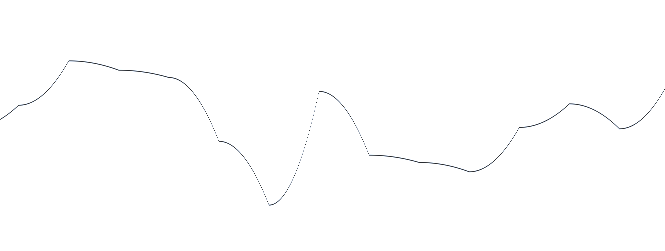With this we can already see how the function is more horizontal just right of the cell positions. The next step is to get a function that can do the same on the left of the cell positions. We’ll call this function EaseOut. For the ease out function we can simply reuse the easeIn function, but instead of pulling low values towards 0, we want to drag values close to 1 closer to 1. To archieve this behaviour, we flip the value, so the values close to 1 are close to 0 and the inverse, then we apply the easeIn function and flip the values again afterwards. We flip the values by simply subtracting them from 1.

``````float easeOut(float interpolator){
return 1 - easeIn(1 - interpolator);
}
``````

The last step to get smooth interpolation is to combine the easing in and easing out. For that we calculate both the ease in and ease out value, then we use the ease in value as the start of the interpolation, near 0, and the ease out value as the end of the interpolation, near 1. the interpolation between the two easing values is a normal linear interpolation like usual.

``````float easeInOut(float interpolator){
float easeInValue = easeIn(interpolator);
float easeOutValue = easeOut(interpolator);
return lerp(easeInValue, easeOutValue, interpolator);
}
``````
``````float interpolator = frac(value);
interpolator = easeInOut(interpolator);
float noise = lerp(previousCellNoise, nextCellNoise, interpolator);
``````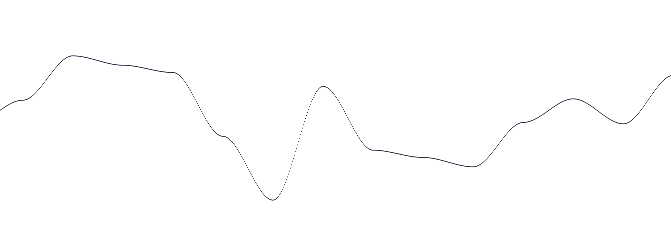And with this we can now smoothly interpolate between the values in 1d.

## Interpolate Cells in two Dimensions

To interpolate two dimensions we choose the 4 closest cells based on the x and y position, then interpolate the ones next to each other on the x axis based on the x fraction and then interpolate that based on the y fraction.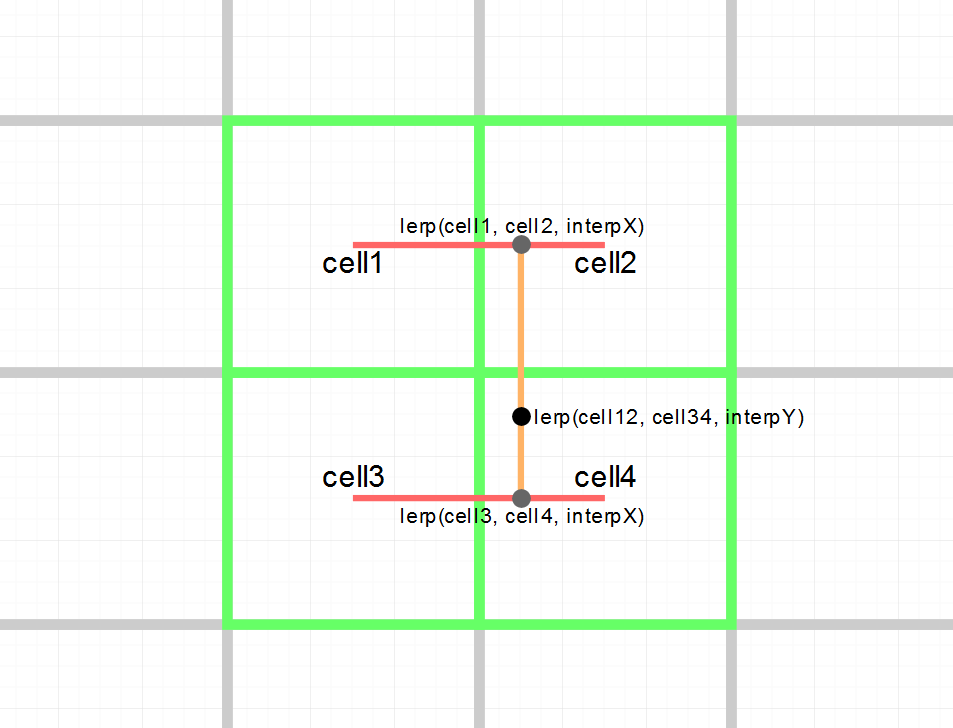Because this is growing into quite a bit of code, we’ll put into it’s own method. To get values to interpolate between in 2d, we’ll use a `rand2dTo[n]d` function. After we generate all 4 cells we need, we generate the interpolation values in the x and y direction including smoothing. Then we generate the interpolated values between the upper and lower cells and finally generate the final value by interpolating between them.

``````float ValueNoise2d(float2 value){
float upperLeftCell = rand2dTo1d(float2(floor(value.x), ceil(value.y)));
float upperRightCell = rand2dTo1d(float2(ceil(value.x), ceil(value.y)));
float lowerLeftCell = rand2dTo1d(float2(floor(value.x), floor(value.y)));
float lowerRightCell = rand2dTo1d(float2(ceil(value.x), floor(value.y)));

float interpolatorX = easeInOut(frac(value.x));
float interpolatorY = easeInOut(frac(value.y));

float upperCells = lerp(upperLeftCell, upperRightCell, interpolatorX);
float lowerCells = lerp(lowerLeftCell, lowerRightCell, interpolatorX);

float noise = lerp(lowerCells, upperCells, interpolatorY);
return noise;
}
``````
``````void surf (Input i, inout SurfaceOutputStandard o) {
float2 value = i.worldPos.xy / _CellSize;
float noise = ValueNoise2d(value);

o.Albedo = noise;
}
``````## Interpolate Cells in three Dimensions and Loops

Interpolating in 3 directions works similarly now, first we read the 8 closest cells, then we interpolate between the pairs that are next to each other on the x axis, then we interpolate between those sets along the y axes so we get 2 values we can then interpolate along the z axis yielding us a single value we can then use to do cool effects with.

But doing this the same way we did the 2d noise results in a lot of code that’s pretty hard to understand and keep in your mind. So to avoid that we’ll use loops. Each loop will only run for 2 iterations (to interpolate between 2 cells at a time). The innermost loop will just read two values which are next to each other in the x axis and save both of them in a short array. After the loop has terminated we can then interpolate between the two values. We add a `[unroll]` attribute before each for loop to make sure the compiler won’t actually execute the code as a loop on the GPU, which can be pretty slow, but instead copies the code of each iteration behind each other.

``````float interpolatorX = easeInOut(frac(value.x));

int y = 0, z = 0;

float cellNoiseX;
[unroll]
for(int x=0;x<=1;x++){
float3 cell = floor(value) + float3(x, y, z);
cellNoiseX[x] = rand3dTo1d(cell);
}
float interpolatedX = lerp(cellNoiseX, cellNoiseX, interpolatorX);
``````

We then wrap a new loop outside of this loop, it will execute the loop which is reading the x neighbors twice and save their results in a new array. After that outer loop is done we can interpolate between the values it wrote into the array to get noise interpolated in 2 dimensions. this is similar to what we did for 2d noise.

``````float interpolatorX = easeInOut(frac(value.x));
float interpolatorY = easeInOut(frac(value.y));

int z = 0;

float cellNoiseY;
[unroll]
for(int y=0;y<=1;y++){
float cellNoiseX;
[unroll]
for(int x=0;x<=1;x++){
float3 cell = floor(value) + float3(x, y, z);
cellNoiseX[x] = rand3dTo1d(cell);
}
cellNoiseY[y] = lerp(cellNoiseX, cellNoiseX, interpolatorX);
}
float interpolatedXY = lerp(cellNoiseY, cellNoiseY, interpolatorY);
``````

And finally we’ll add a final loop around the existing ones, in this one we’ll execute the loop reading a pair in the y direction twice (which in turn will execute the loop reading a pair in the x direction twice, executing the innermost code 8 times, once for each cell). Just like the inner loops it will also write the result into a tiny array so that after it’s done, we can interpolate in the z direction and get our final value.

``````float ValueNoise3d(float3 value){
float interpolatorX = easeInOut(frac(value.x));
float interpolatorY = easeInOut(frac(value.y));
float interpolatorZ = easeInOut(frac(value.z));

float cellNoiseZ;
[unroll]
for(int z=0;z<=1;z++){
float cellNoiseY;
[unroll]
for(int y=0;y<=1;y++){
float cellNoiseX;
[unroll]
for(int x=0;x<=1;x++){
float3 cell = floor(value) + float3(x, y, z);
cellNoiseX[x] = rand3dTo1d(cell);
}
cellNoiseY[y] = lerp(cellNoiseX, cellNoiseX, interpolatorX);
}
cellNoiseZ[z] = lerp(cellNoiseY, cellNoiseY, interpolatorY);
}
float noise = lerp(cellNoiseZ, cellNoiseZ, interpolatorZ);
return noise;
}
``````
``````void surf (Input i, inout SurfaceOutputStandard o) {
float3 value = i.worldPos.xyz / _CellSize;
float noise = ValueNoise3d(value);

o.Albedo = noise;
}
``````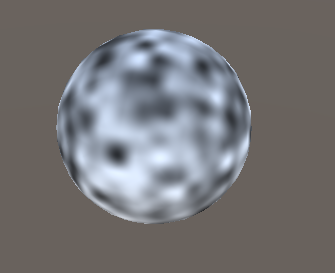## 3d Output Values

Once we have the function it’s pretty easy to change it so it doesn’t just return greyscale values, but colorful values instead. We simply have to use the rand3dTo3d function to get the appropriate colorful values. Then we also have to change the datatype of all of the arrays, return value and all other values where we save the noise to the datatype we want to return, so float3 in our case.

``````float3 ValueNoise3d(float3 value){
float interpolatorX = easeInOut(frac(value.x));
float interpolatorY = easeInOut(frac(value.y));
float interpolatorZ = easeInOut(frac(value.z));

float3 cellNoiseZ;
[unroll]
for(int z=0;z<=1;z++){
float3 cellNoiseY;
[unroll]
for(int y=0;y<=1;y++){
float3 cellNoiseX;
[unroll]
for(int x=0;x<=1;x++){
float3 cell = floor(value) + float3(x, y, z);
cellNoiseX[x] = rand3dTo3d(cell);
}
cellNoiseY[y] = lerp(cellNoiseX, cellNoiseX, interpolatorX);
}
cellNoiseZ[z] = lerp(cellNoiseY, cellNoiseY, interpolatorY);
}
float3 noise = lerp(cellNoiseZ, cellNoiseZ, interpolatorZ);
return noise;
}
``````
``````void surf (Input i, inout SurfaceOutputStandard o) {
float3 value = i.worldPos.xyz / _CellSize;
float3 noise = ValueNoise3d(value);

o.Albedo = noise;
}
``````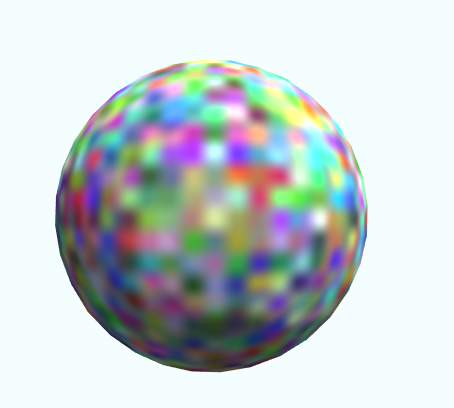## Source

``````Shader "Tutorial/025_value_noise/1d" {
Properties {
_CellSize ("Cell Size", Range(0, 1)) = 1
}
Tags{ "RenderType"="Opaque" "Queue"="Geometry"}

CGPROGRAM

#pragma target 3.0

#include "Random.cginc"

float _CellSize;

struct Input {
float3 worldPos;
};

float easeIn(float interpolator){
return interpolator * interpolator;
}

float easeOut(float interpolator){
return 1 - easeIn(1 - interpolator);
}

float easeInOut(float interpolator){
float easeInValue = easeIn(interpolator);
float easeOutValue = easeOut(interpolator);
return lerp(easeInValue, easeOutValue, interpolator);
}

void surf (Input i, inout SurfaceOutputStandard o) {
float value = i.worldPos.x / _CellSize;
float previousCellNoise = rand1dTo1d(floor(value));
float nextCellNoise = rand1dTo1d(ceil(value));
float interpolator = frac(value);
interpolator = easeInOut(interpolator);
float noise = lerp(previousCellNoise, nextCellNoise, interpolator);

float dist = abs(noise - i.worldPos.y);
float pixelHeight = fwidth(i.worldPos.y);
float lineIntensity = smoothstep(0, pixelHeight, dist);
o.Albedo = lineIntensity;
}
ENDCG
}
FallBack "Standard"
}
``````
``````Shader "Tutorial/025_value_noise/2d" {
Properties {
_CellSize ("Cell Size", Range(0, 1)) = 1
}
Tags{ "RenderType"="Opaque" "Queue"="Geometry"}

CGPROGRAM

#pragma target 3.0

#include "Random.cginc"

float _CellSize;

struct Input {
float3 worldPos;
};

float easeIn(float interpolator){
return interpolator * interpolator;
}

float easeOut(float interpolator){
return 1 - easeIn(1 - interpolator);
}

float easeInOut(float interpolator){
float easeInValue = easeIn(interpolator);
float easeOutValue = easeOut(interpolator);
return lerp(easeInValue, easeOutValue, interpolator);
}

float ValueNoise2d(float2 value){
float upperLeftCell = rand2dTo1d(float2(floor(value.x), ceil(value.y)));
float upperRightCell = rand2dTo1d(float2(ceil(value.x), ceil(value.y)));
float lowerLeftCell = rand2dTo1d(float2(floor(value.x), floor(value.y)));
float lowerRightCell = rand2dTo1d(float2(ceil(value.x), floor(value.y)));

float interpolatorX = easeInOut(frac(value.x));
float interpolatorY = easeInOut(frac(value.y));

float upperCells = lerp(upperLeftCell, upperRightCell, interpolatorX);
float lowerCells = lerp(lowerLeftCell, lowerRightCell, interpolatorX);

float noise = lerp(lowerCells, upperCells, interpolatorY);
return noise;
}

void surf (Input i, inout SurfaceOutputStandard o) {
float2 value = i.worldPos.xy / _CellSize;
float noise = ValueNoise2d(value);

o.Albedo = noise;
}
ENDCG
}
FallBack "Standard"
}
``````
``````Shader "Tutorial/025_value_noise/3d" {
Properties {
_CellSize ("Cell Size", Range(0, 1)) = 1
}
Tags{ "RenderType"="Opaque" "Queue"="Geometry"}

CGPROGRAM

#pragma target 3.0

#include "Random.cginc"

float _CellSize;

struct Input {
float3 worldPos;
};

float easeIn(float interpolator){
return interpolator * interpolator;
}

float easeOut(float interpolator){
return 1 - easeIn(1 - interpolator);
}

float easeInOut(float interpolator){
float easeInValue = easeIn(interpolator);
float easeOutValue = easeOut(interpolator);
return lerp(easeInValue, easeOutValue, interpolator);
}

float3 ValueNoise3d(float3 value){
float interpolatorX = easeInOut(frac(value.x));
float interpolatorY = easeInOut(frac(value.y));
float interpolatorZ = easeInOut(frac(value.z));

float3 cellNoiseZ;
[unroll]
for(int z=0;z<=1;z++){
float3 cellNoiseY;
[unroll]
for(int y=0;y<=1;y++){
float3 cellNoiseX;
[unroll]
for(int x=0;x<=1;x++){
float3 cell = floor(value) + float3(x, y, z);
cellNoiseX[x] = rand3dTo3d(cell);
}
cellNoiseY[y] = lerp(cellNoiseX, cellNoiseX, interpolatorX);
}
cellNoiseZ[z] = lerp(cellNoiseY, cellNoiseY, interpolatorY);
}
float3 noise = lerp(cellNoiseZ, cellNoiseZ, interpolatorZ);
return noise;
}

void surf (Input i, inout SurfaceOutputStandard o) {
float3 value = i.worldPos.xyz / _CellSize;
float3 noise = ValueNoise3d(value);

o.Albedo = noise;
}
ENDCG
}
FallBack "Standard"
}
``````

You can also find the source on github:

I hope this tutorial helped you understand how to interpolate between random values to generate smoother patterns.

You can also find me on twitter at @totallyRonja. If you liked my tutorial and want to support me you can do that on Patreon (patreon.com/RonjaTutorials) or Ko-Fi (ko-fi.com/RonjaTutorials).